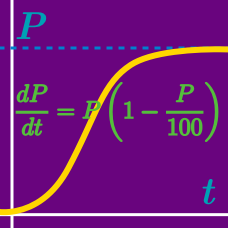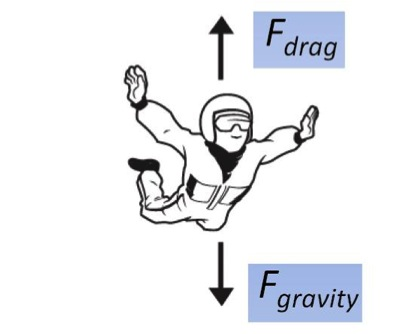Calculus

# First Order Differential Equations: Level 2 ChallengesTwo forces act on a parachutist. One is $mg,$ the attraction by the earth, where $m$ is the mass of the person plus equipment and $g=9.8 \text{ m/sec}^2$ is the acceleration of gravity. The other force is the air resistance ("drag"), which is assumed to be proportional to the square of the velocity $v(t)$.

Using Newton's second law of motion (mass $\times$ acceleration = net force applied), set up an ordinary differential equation for $v(t).$

Let $k$ denote the drag coefficient.You have a faulty laptop battery whose charge gauge acts very strangely. Particularly, after every minute, its estimate of how much time you have left before it dies drops by $d$ minutes, where $d$ is a positive number. The gauge estimates the rate at which you deplete the battery by finding the average rate of change of your battery charge thus far with respect to time. Your battery takes exactly $m$ minutes to die. The function $f(t)$, where $t$ is a positive number of minutes, describes the amount of battery charge used (i.e. $f(0) = 0$ when your battery is charged, and $f(m) = 1$ when your battery is dead). Which is the correct form of $f(t)$?

You are given the differential equation $\frac{dy}{dx} + y \ln x = x^{-x} e^{-x}$ and the initial condition $y(1) = 0$

If the value of $y(2)$ can be written in the form $\dfrac{e^{2}+a}{be^2}$ determine the value of $a + b$.

Solve the ODE $xy'+y={(xy)}^{2} \ln{x}$ where $y(1)=\frac{1}{3}$. Calculate the value of $y(e)$ to 3 decimal places.

What is the order of the differential equation $x^2.\left( \dfrac{d^2y}{dx^2} \right)^6+y^{\frac{-2}{3}}.\sqrt{1+\left( \dfrac{d^3y}{dx^3} \right)^5}+\dfrac{d^2}{dx^2}. \left( \dfrac{d^2y}{dx^2} \right)^{\frac{-2}{3}} =0?$

×